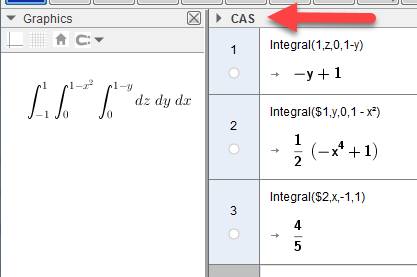# Creating a custom 3D solid

Binza shared this question 2 years ago

Hello,

So I want to create a curved solid at is bounded by different planes,

Eg. Solid A is bounded by:

z = 1 - y^2

1 = x + z

0 = x

0 = z

How do I make GeoGebra interpret it as a solid, so I can take its volume for example?1

try changing

x--->z; y---->x; z---->y

then xmin=-1 xmax=1 ymin=0 ymax=1-x^2 zmin=0 zmax=1-y in my applet